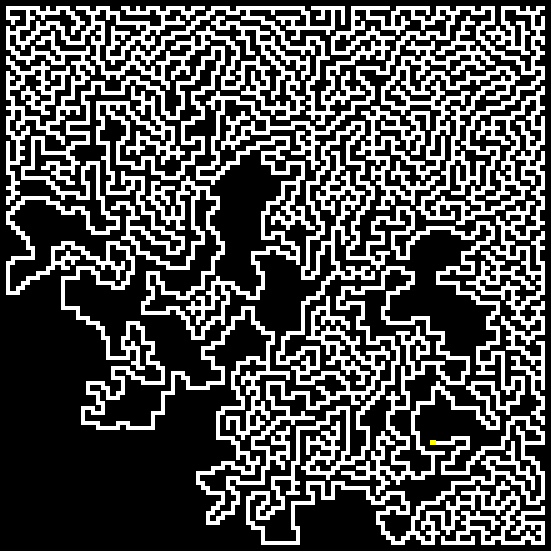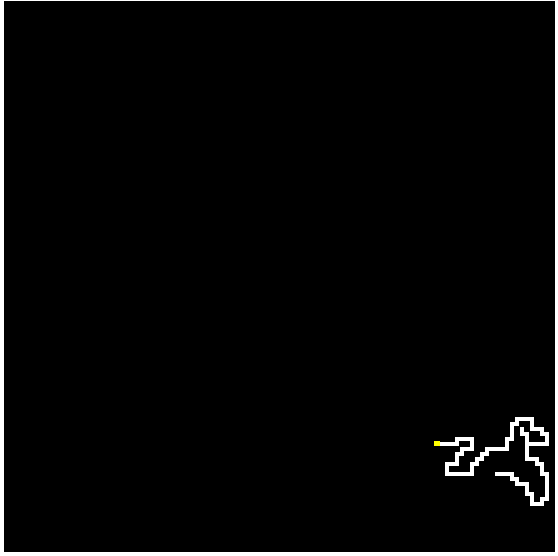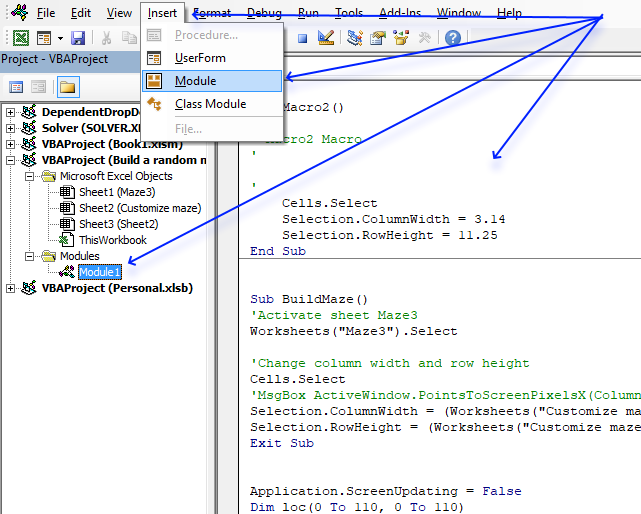Author: Oscar Cronquist Article last updated on November 11, 2020The image above shows the creation of a maze located on a worksheet. A macro builds this maze randomly, a new version is generated each time you start the macro.

Two weeks ago I posted a link to a workbook containing formulas calculating the shortest path in a maze. Today I have created a macro that builds a random maze.

The cell grid is 110 x 110 and the start cell is randomly chosen and colored yellow. The macro then randomly creates a path until it can´t move further. The endpoint is a cell with the longest distance to the start cell, colored blue.

The path between them is usually around 2500 cells. You can find the blue cell somewhere in the upper left corner, at the end of the animation. There is only one possible path between the start cell and end cell.

The animated image below shows the macro creating the maze, you can see how the macro creates new paths when there is nowhere to go.To start building a new maze press with left mouse button on the button to the right of the maze, on the worksheet.

### Change animation speed

You can change the speed of this by changing this line in the macro:

```If (k / 100) - Int(k / 100) = 0 Then
```

If you want it really slow, change it to:

```If (k / 1) - Int(k / 1) = 0 Then
```

If you want it faster, change it to:

```If (k / 200) - Int(k / 200) = 0 Then
```

### Count distance between start cell and end cell

```MsgBox Tcount
```

before End Sub

### VBA Code

```'Name macro
Sub BuildMaze()

'Disable screen refresh
Application.ScreenUpdating = False

'Dimension variables and declare data types
'Array variable loc keeps track of the maze in creation
Dim loc(0 To 110, 0 To 110)

'Array variable path keeps track of places to go not visited earlier
Dim path(0 To 3)

'The visloc array variable keeps track of the current coordinate
Dim visloc() As Variant

'Redimension array making it a dynamic array
ReDim visloc(1, 0)

'Save value 1 to each cell in cell range B2:DG111
Range("B2:DG111") = 1

'Create a random value between 1 and 108 and save it ti variables StartR and StartC
StartR = Int(Rnd * 108) + 1
StartC = Int(Rnd * 108) + 1

'Save text value S to a cell in cell range B2:DG111 based on random values stored in variables StartR and StartC
Range("B2:DG111").Cells(StartR, StartC) = "S"

'Save number 1 to array variable loc based on random values stored in variables StartR and StartC
loc(StartR, StartC) = 1

'Save random values stored in variables StartR and StartC to array variable visloc row 0 (zero), column 0 (zero) and row 1 and column 0 (zero)
visloc(0, 0) = StartR
visloc(1, 0) = StartC

'Do ... Loop statement until a condition is met meaning the lines between Do and Loop until will be rund repeatedly until the condition is met
Do

'Save 0 (zero) to variable c
c = 0

'For ... Next statement repeats line(s) between For and Next 4 times (0, 1, 2, 3)
For i = 0 To 3

'Save zero to array variable path based on variable i
path(i) = 0
Next i

'If ... then statement runs lines between If and End If if a condition is met
If visloc(0, UBound(visloc, 2)) - 2 >= 1 Then

'If ... then statement runs lines between If and End If if the conditions are met
If loc(visloc(0, UBound(visloc, 2)) - 1, visloc(1, UBound(visloc, 2))) <> 1 And _
loc(visloc(0, UBound(visloc, 2)) - 2, visloc(1, UBound(visloc, 2))) <> 1 And _
loc(visloc(0, UBound(visloc, 2)) - 1, visloc(1, UBound(visloc, 2)) + 1) <> 1 And _
loc(visloc(0, UBound(visloc, 2)) - 1, visloc(1, UBound(visloc, 2)) - 1) <> 1 Then

'Save number 1 to array variable path position 0
path(0) = 1

'Save number 1 to variable c
c = 1
End If
End If

'If ... then statement runs lines between If and End If if a condition is met
If visloc(0, UBound(visloc, 2)) + 2 <= 110 Then

'If ... then statement runs lines between If and End If if the conditions are met
If loc(visloc(0, UBound(visloc, 2)) + 1, visloc(1, UBound(visloc, 2))) <> 1 And _
loc(visloc(0, UBound(visloc, 2)) + 2, visloc(1, UBound(visloc, 2))) <> 1 And _
loc(visloc(0, UBound(visloc, 2)) + 1, visloc(1, UBound(visloc, 2)) + 1) <> 1 And _
loc(visloc(0, UBound(visloc, 2)) + 1, visloc(1, UBound(visloc, 2)) - 1) <> 1 Then

'Save number 1 to variable c
c = 1

'Save number 1 to array variable path position 1
path(1) = 1
End If
End If

'If ... then statement runs lines between If and End If if a condition is met
If visloc(1, UBound(visloc, 2)) - 2 >= 1 Then

'If ... then statement runs lines between If and End If if the conditions are met
If loc(visloc(0, UBound(visloc, 2)), visloc(1, UBound(visloc, 2)) - 1) <> 1 And _
loc(visloc(0, UBound(visloc, 2)), visloc(1, UBound(visloc, 2)) - 2) <> 1 And _
loc(visloc(0, UBound(visloc, 2)) + 1, visloc(1, UBound(visloc, 2)) - 1) <> 1 And _
loc(visloc(0, UBound(visloc, 2)) - 1, visloc(1, UBound(visloc, 2)) - 1) <> 1 Then

'Save number 1 to variable c
c = 1

'Save number 1 to array variable path position 2
path(2) = 1
End If
End If

'If ... then statement runs lines between If and End If if a condition is met
If visloc(1, UBound(visloc, 2)) + 2 <= 110 Then

'If ... then statement runs lines between If and End If if the conditions are met
If loc(visloc(0, UBound(visloc, 2)), visloc(1, UBound(visloc, 2)) + 1) <> 1 And _
loc(visloc(0, UBound(visloc, 2)), visloc(1, UBound(visloc, 2)) + 2) <> 1 And _
loc(visloc(0, UBound(visloc, 2)) + 1, visloc(1, UBound(visloc, 2)) + 1) <> 1 And _
loc(visloc(0, UBound(visloc, 2)) - 1, visloc(1, UBound(visloc, 2)) + 1) <> 1 Then

'Save number 1 to variable c
c = 1

'Save number 1 to array variable path position 3
path(3) = 1
End If
End If

'Check if variable c is equal to 0 (zero)
If c = 0 Then

'Check if number saved in variable Ccount is larger than number stored in variable Tcount
If Ccount > Tcount Then

'Save number stored in variable Ccount to variable Tcount
Tcount = Ccount

'Save number stored in array variable visloc in position row 0 and column UBound(visloc, 2)
Er = visloc(0, UBound(visloc, 2))

'Save number stored in array variable visloc in position row 1 and column UBound(visloc, 2)
Ec = visloc(1, UBound(visloc, 2))
End If

'Subtract number stored in variable Ccount with 1
Ccount = Ccount - 1

'Redimension array variable visloc
ReDim Preserve visloc(UBound(visloc, 1), UBound(visloc, 2) - 1)

'Continue here if variable c is not equal to 0 (zero)
Else

'Save 0 (zero) to variable c
c = 0

'Keep iterating until c is not equal to 0 (zero)
Do Until c <> 0

'Create a random integer between 0 and 4
rrand = Int(Rnd * 4)

'Check if number stored in array variable path position based on number in variable rrand is equal to 1
'If True then save number stored in variable rrand plus 1 to variable c
If path(rrand) = 1 Then c = rrand + 1
Loop

'Redimension array variable visloc but keep previous values
ReDim Preserve visloc(UBound(visloc, 1), UBound(visloc, 2) + 1)

'Select case statement. Based on what variable c contains case 1 to 4 is rund
Select Case c

'If variable c is equal to 1
Case 1
visloc(0, UBound(visloc, 2)) = visloc(0, UBound(visloc, 2) - 1) - 1
visloc(1, UBound(visloc, 2)) = visloc(1, UBound(visloc, 2) - 1)

'If variable c is equal to 2
Case 2
visloc(0, UBound(visloc, 2)) = visloc(0, UBound(visloc, 2) - 1) + 1
visloc(1, UBound(visloc, 2)) = visloc(1, UBound(visloc, 2) - 1)

'If variable c is equal to 3
Case 3
visloc(0, UBound(visloc, 2)) = visloc(0, UBound(visloc, 2) - 1)
visloc(1, UBound(visloc, 2)) = visloc(1, UBound(visloc, 2) - 1) - 1

'If variable c is equal to 4
Case 4
visloc(0, UBound(visloc, 2)) = visloc(0, UBound(visloc, 2) - 1)
visloc(1, UBound(visloc, 2)) = visloc(1, UBound(visloc, 2) - 1) + 1
End Select

'Add 1 to number stored in variable Ccount
Ccount = Ccount + 1

'Clear values in cell range B2:DG111
Range("B2:DG111").Cells(visloc(0, UBound(visloc, 2)), visloc(1, UBound(visloc, 2))) = ""

'Save number 1 to array variable visloc
loc(visloc(0, UBound(visloc, 2)), visloc(1, UBound(visloc, 2))) = 1

'Apply changes
DoEvents
End If

k = k + 1

'If k is 50, 100, 150 and so on ...
If (k / 50) - Int(k / 50) = 0 Then

'Show changes on screen
Application.ScreenUpdating = True

'Hide changes on screen
Application.ScreenUpdating = False
End If

'Loop until array variable visloc is equal to start coordinates meaning the maze is complete
Loop Until visloc(0, UBound(visloc, 2)) = StartR And visloc(1, UBound(visloc, 2)) = StartC

'Show changes on screen
Application.ScreenUpdating = True

'Save text value B to cell in cell range B2:DG111 based on coordinates in variable Er and Ec
Range("B2:DG111").Cells(Er, Ec) = "B"

End Sub
```

### Where to put the code?1. Press shortcut keys Alt + F11 to open the Visual Basic Editor (VB Editor).
2. Press with left mouse button on "Insert" on the top menu, see image above.
3. Press with mouse on "Module" to create a module in your workbook. The image above shows the module named Module1 in the Project Explorer window.
4. Copy and paste VBA code to the module which is also shown in the image above.